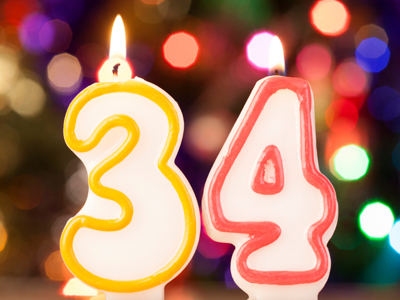The best method of multiplying 34 by 4 is to double and double again.

# Multiplication and Division 2

This Math quiz is called 'Multiplication and Division 2' and it has been written by teachers to help you if you are studying the subject at elementary school. Playing educational quizzes is an enjoyable way to learn if you are in the 3rd, 4th or 5th grade - aged 8 to 11.

It costs only \$12.50 per month to play this quiz and over 3,500 others that help you with your school work. You can subscribe on the page at Join Us

If you want to find the product of two numbers then multiply them together. The numbers that we can divide a number by are it's factors. Products and factors are just two parts of multiplication and division. Multiplication and division are often used by people in their everyday life, especially when going shopping! Three children have broken their toothbrushes so their Mom is buying three new ones. They cost \$4 each, so to find the total she has to multiply \$4 by 3. If Mom knows the answer then she knows just how much she is spending.

See how much you know about multiplication and division by playing this quiz.

1.
What is the product of 3 and 6?
2
3
9
18
To find the product of two numbers they have to be multiplied together
2.
What is the best method of multiplying 34 by 4?
Double and double again
Multiply by 3 twice
Multiply by 3 then halve
Multiply by 2 then halve
Double twice to multiply any number by 4
3.
How do you find half of a number?
Multiply it by 4
Divide it by 2
Multiply it by 2
Divide it by 4
Half means the same as divided by 2
4.
Which of these is not equivalent to 64 x 3?
64 + 64 + 64
3 x 64
6 x 64 x 2
64 x 2 + 64
The correct answer is 192 but 6 x 64 x 2 = 768
5.
Which strategy would be best to multiply 87 by 5?
Multiply by 4 then add another 87
Double then multiply by 3
Multiply by 10 then halve
Multiply by 3 then halve
10 and 2 are easier numbers to deal with than 5 is
6.
12 x 0 = ?
0
1
6
12
Any number multiplied by 0 will give the answer 0
7.
If we divide 10 by 4 what is the answer?
2
2 remainder 2
It can’t be done
3
2 x 4 = 8 so there are 2 numbers left over
8.
Which times table would you have if you doubled the 4 times table?
The 2 times table
The 3 times table
The times table
The 8 times table
2 x 4 = 8
9.
Which is equivalent to multiply by 10 then double?
x 5
x 15
x 20
x 25
Remember that double means the same as x 2, so 10 x 2 = 20
10.
How do you find quarter of a number?
Multiply it by 4
Divide it by 2
Multiply it by 2
Divide it by 4
Quarter means the same as divided by 4
Author:  Amanda Swift